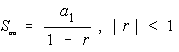SERIES Program

A menu driven program which contains a collection of algorithms for sequences and series from a College Algebra level course.

You can use it to:

• Find the general term of an arithmetic sequence when the first term, common difference, and number of terms are known.
• Find the sum of an arithmetic sequence when the first and last terms are known.
• Find the sum of an arithmetic sequence when the first term, common difference, and number of terms are known.
• Find the general term of a geometric sequence when the first term, common ratio, and number of terms are known.
• Find the sum of a geometric sequence when the first term, common ratio, and number of terms are known.
• Find the sum of an infinite geometric sequence when the first term, common ratio, and number of terms are known.

Formulas

General Term of an Arithmetic Sequence: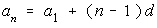Arithmetic Series: (two formulas)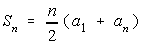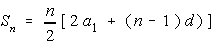General Term of a Geometric Sequence: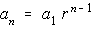Geometric Series: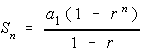Infinite Geometric Series: